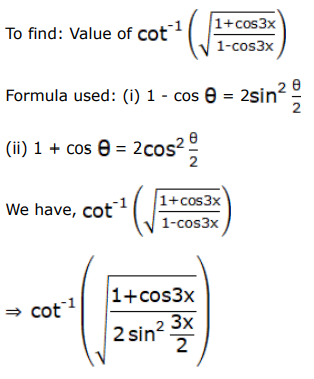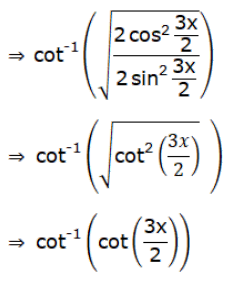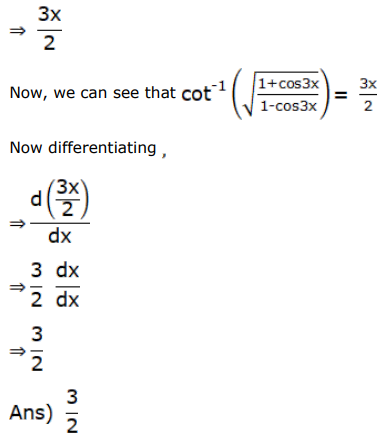# Differentiate each of the following w.r.tQuestion:

Differentiate each of the following w.r.t $x$ :

$\cot ^{-1}\left(\sqrt{\frac{1+\cos 3 x}{1-\cos 3 x}}\right)$

Solution: